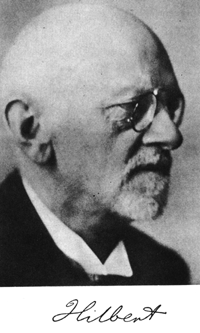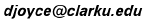# Hilbert's Mathematical Problems

(The actual text is on a separate page.)

Introduction. Problem 1. (Philosophy of problems, relationship between mathematics and science, role of proofs, axioms and formalism.) Cantor's problem of the cardinal number of the continuum. (The continuum hypothesis.) K. Gödel. The consistency of the axiom of choice and of the generalized continuum hypothesis. Princeton Univ. Press, Princeton, 1940. The compatibility of the arithmetical axioms. The equality of two volumes of two tetrahedra of equal bases and equal altitudes. V. G. Boltianskii. Hilbert's Third Problem Winston, Halsted Press, Washington, New York, 1978. C. H. Sah. Hilbert's Third Problem: Scissors Congruence. Pitman, London 1979. Problem of the straight line as the shortest distance between two points. (Alternative geometries.) Lie's concept of a continuous group of transformations without the assumption of the differentiability of the functions defining the group. (Are continuous groups automatically differential groups?) Montgomery and Zippin. Topological Transformation Groups. Wiley, New York, 1955. Kaplansky. Lie Algebras and Locally Compact Groups. Chicago Univ. Press, Chicago, 1971. Mathematical treatment of the axioms of physics. Leo Corry's article "Hilbert and the Axiomatization of Physics (1894-1905)" in the research journal Archive for History of Exact Sciences, 51 (1997). Irrationality and transcendence of certain numbers. N.I.Feldman. Hilbert's seventh problem (in Russian), Moscow state Univ, 1982, 312pp. MR 85b:11001 Problems of prime numbers. (The distribution of primes and the Riemann hypothesis.) Proof of the most general law of reciprocity in any number field. Determination of the solvability of a diophantine equation. S. Chowla. The Riemann Hypothesis and Hilbert's Tenth Problem. Gordon and Breach, New York, 1965. Yu. V. Matiyasevich. Hilbert's Tenth Problem. MIT Press, Cambridge, Massachusetts,1993, available on the web. Maxim Vsemirnov's Hilbert's Tenth Problem page at the Steklov Institute of Mathematics at St.Petersburg. Quadratic forms with any algebraic numerical coefficients. Extension of Kroneker's theorem on abelian fields to any algebraic realm of rationality. R.-P. Holzapfel. The Ball and Some Hilbert Problems. Springer-Verlag, New York, 1995. Impossibility of the solution of the general equation of the 7-th degree by means of functions of only two arguments. (Generalizes the impossibility of solving 5-th degree equations by radicals.) Proof of the finiteness of certain complete systems of functions. Masayoshi Nagata. Lectures on the fourteenth problem of Hilbert. Tata Institute of Fundamental Research, Bombay, 1965. Rigorous foundation of Schubert's enumerative calculus. Problem of the topology of algebraic curves and surfaces. Yu. Ilyashenko, and S. Yakovenko, editors. Concerning the Hilbert 16th problem. American Mathematical Society, Providence, R.I., 1995. B.L.J. Braaksma, G.K. Immink, and M. van der Put, editors. The Stokes Phenomenon and Hilbert's 16th Problem. World Scientific, London, 1996. Expression of definite forms by squares. Building up of space from congruent polyhedra. (n-dimensional crystallography groups, fundamental domains, sphere packing problem.) on the theory of analytic functions. Are the solutions of regular problems in the calculus of variations always necessarily analytic? The general problem of boundary values. (Variational problems.) Proof of the existence of linear differential equations having a prescribed monodromic group. Uniformization of analytic relations by means of automorphic functions. Further development of the methods of the calculus of variations.

March, 1997.
David E. Joyce
Department of Mathematics and Computer Science
Clark University
Worcester, MA 01610These files are located at http://aleph0.clarku.edu/~djoyce/hilbert/